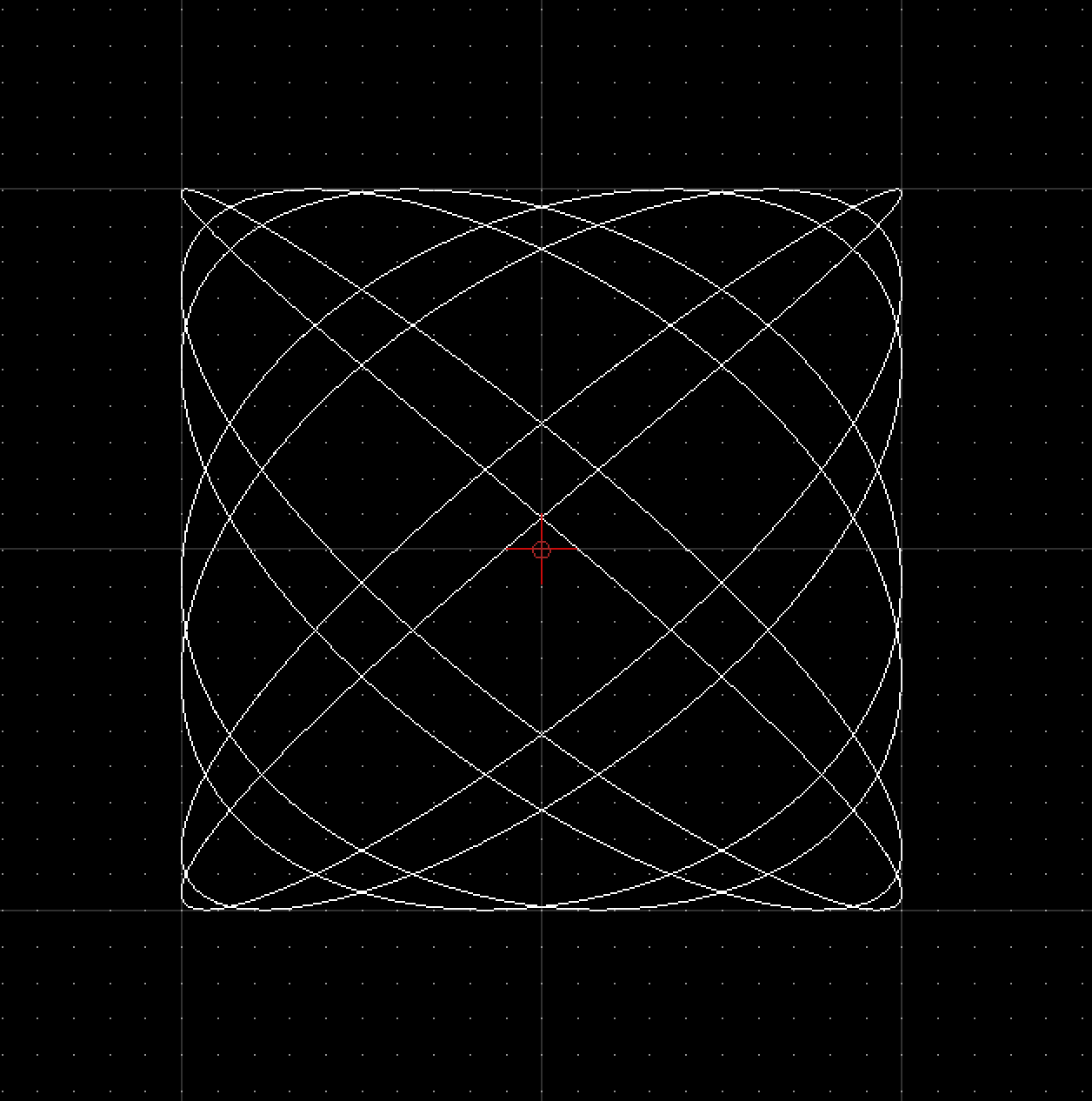# Tutorial: Running Scripts from a File

## Introduction

It can quickly become tedious to type scripts into the Script Shell of QCAD. As soon as scripts are getting slightly more complex, you might want to write them into a file instead. For procedural scripts which do not require interaction, you can simply create a text file with calls to the QCAD API and run the script using Misc > Development > Run Script.

## Examples

### Plotting Functions

A typical task for a script would be to plot a mathematical function. This example plots a Lissajous curve.

Note that the lines starting with // are comments. Comments are ignored and are purely intended for explanation.

 ``` 1 2 3 4 5 6 7 8 9 10 11 12 13 14 15 16 17 18 19 20 21 22 23 24 25 26 27 28 29 30``` ```// constants: a=7; b=6; // phase angle: phi=0.2*Math.PI; // iteration step: step=Math.PI/256; // width of plot (amplitude in X): w=100; // height of plot (amplitude in Y): h=100; // array of x,y coordinates on the curve: v=[]; // loop: for (t=0.0; t